# 大数据Spark MLlib推荐算法

+关注继续查看

## 1 相似度算法

• 欧几里德距离算法（Euclidean Distance）
• 皮尔逊相似度算法（Pearson Correlation Coefficient）
• 基于夹角余弦相似度算法（Consine Similarity）
• 基于Tanimoto系数相似度（Tanimoto Coefficient）

### 1.1 欧几里德距离算法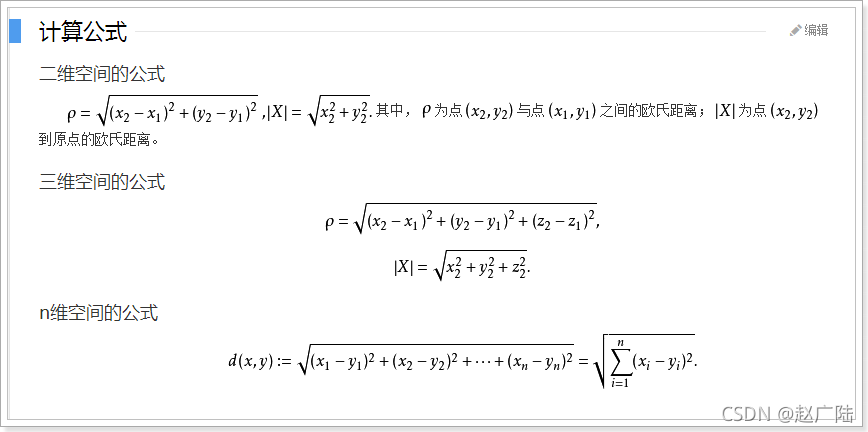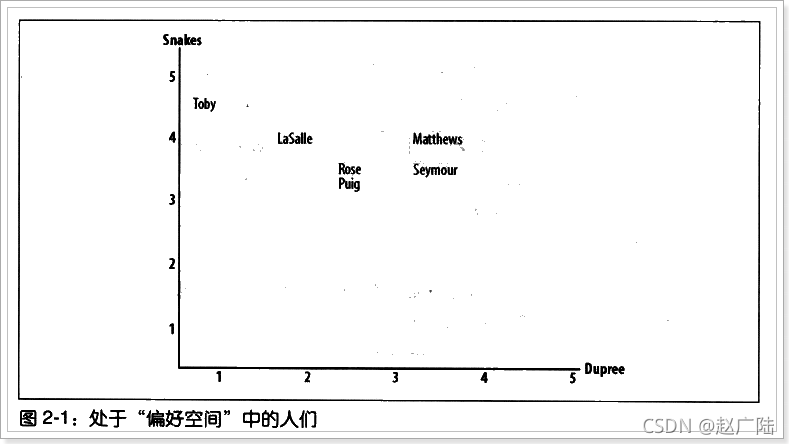### 1.2 基于夹角余弦相似度算法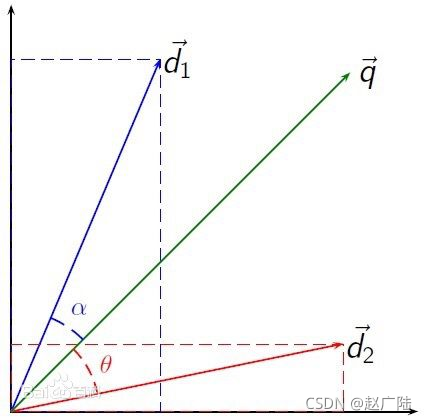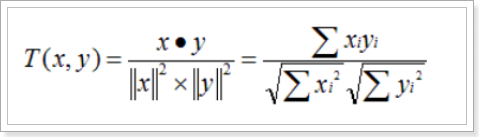## 2 最近邻域

• 固定数量的邻居：K-neighborhoods
• 基于相似度门槛的邻居：Threshold-based neighborhoods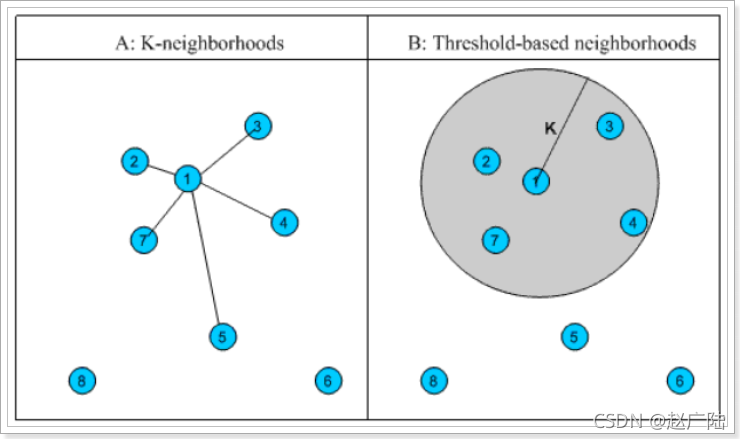## 3 交替最小二乘法

### 3.1 最小二乘法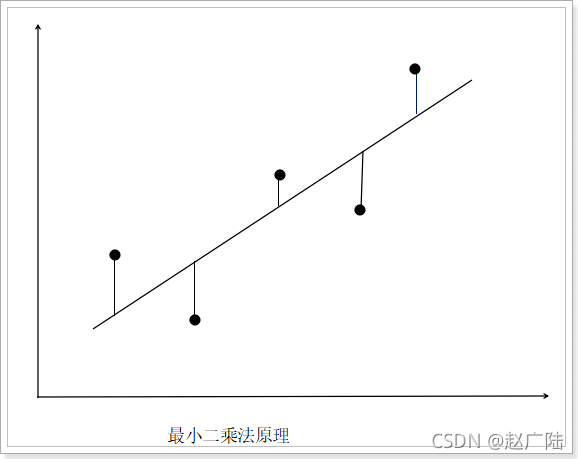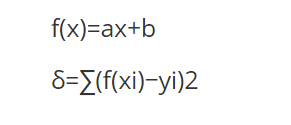2是开方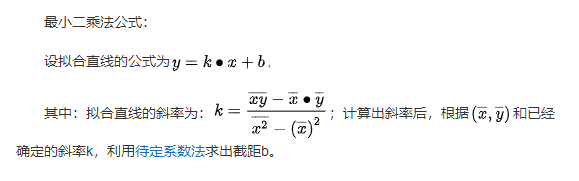### 3.2 交替最小二乘法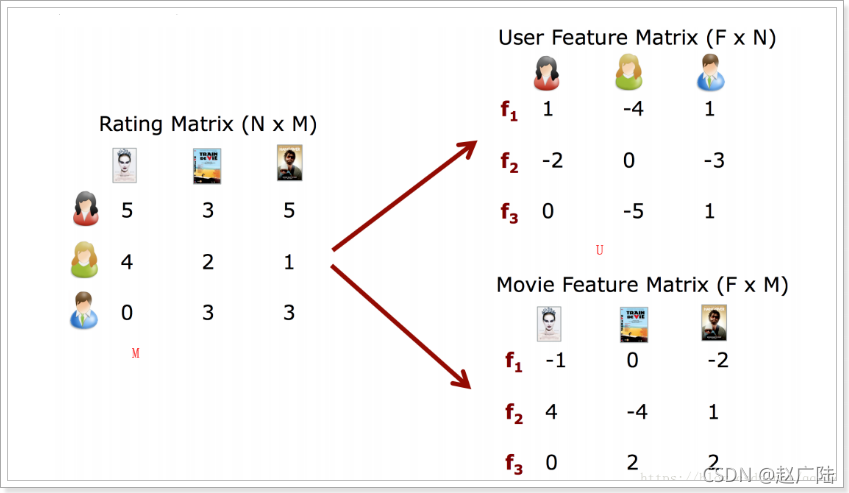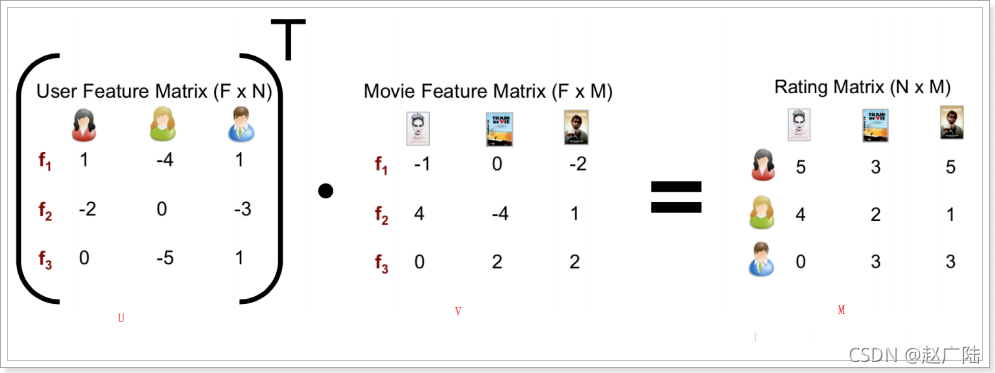### 3.3 ALS算法流程

ALS算法解决了用户评分矩阵中的缺失因子问题，实现了用预测得到的缺失因子进行推荐。

ALS 算法的思路就不同了。 ALS 算法基于下面这个假设：评分矩阵是近似低秩 (Low・Rank) 矩阵；换句话说，评分矩阵 A(mxn) 可以用两个小矩阵 U(mxk) 和 V(nxk) 来近似表示,即：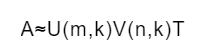ALS 算法的低秩假设是建立在客观存在的合理性基础上的。例如，用户特征有很多，如年龄、性别、职业、身高、学历、婚姻、地区、存款等，可以说不胜枚举，但我们没有必要把用户的所有特征都用起来，因为并不是所有特征都起同样的作用。例如，在后面展示的电影推荐示例中，用户特征矩阵仅仅包含了用户编号、性别、年龄、职业、邮编5 个字段。同样，物品的属性也有很多，以电影为例，可以有主演、导演、特效、剧情、类型等，但实际应用中我们只需要描述少数关键属性即可，因此我们仅仅考虑了三个属性，即电影编号、电影名和电影类别(当然这只是示例，到底 k 取什么值，可以采用系统自适应调节方法，通过应用逐步找到最佳的 k 值)。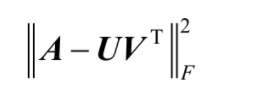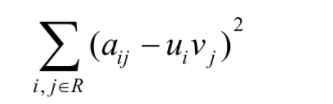ALS 算法可以大体描述如下。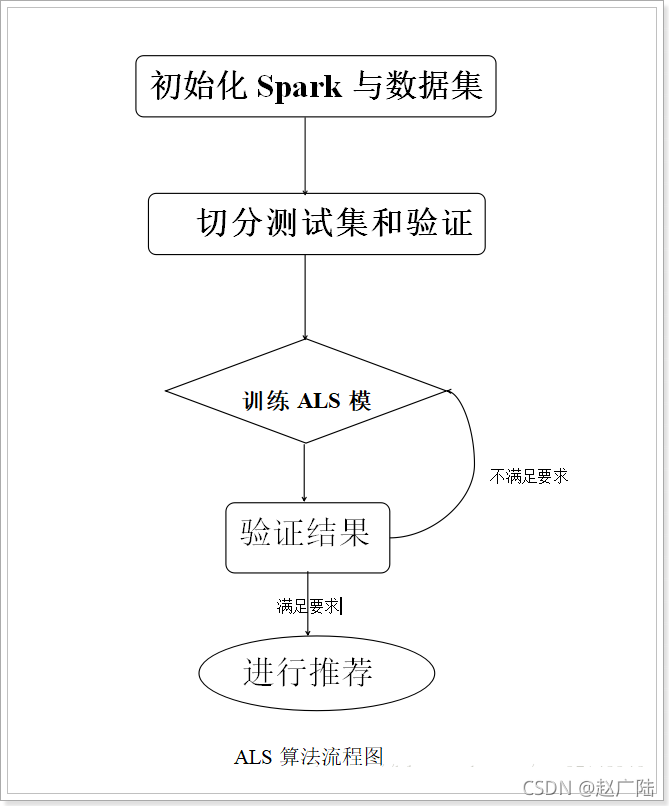### 3.4 ALS算法实战

#### 3.4.1 数据说明

ALS 算法对 GroupLens Research （ http://grouplens.org/datasets/movielens/） 提供的数据进行学习并推荐。该数据为一组从 20 世纪 90 年代末到 21 世纪初由 MovieLens 用户提供的电影评价数据，包括评分、电影元数据（如风格类型和年代），以及关于用户的人口统计学数据（如年龄、邮编、性别和职业等）。根据不同需求， GroupLens Research 提供了不同大小的样本数据，包含了评分、用户信息和电影信息三种数据。下面先来看看待处理的电影评价数据，再给出应用程序并进行分析。

196 242 3   881250949
186 302 3   891717742
22  377 1   878887116
244 51  2   880606923
166 346 1   886397596
298 474 4   884182806
115 265 2   881171488
253 465 5   891628467
305 451 3   886324817
6   86  3   883603013
62  257 2   879372434
286 1014    5   879781125
200 222 5   876042340
210 40  3   891035994
................


#### 3.4.2 数据建模

ALS算法的第二步就是数据建模，其实在MLlib算法库中有可以直接使用的训练算法，ALS.tran方法源码如下：

 def train(
ratings: RDD[Rating],       //需要训练的数据集
rank: Int,                  //模型中隐藏因子数，rank一般选在8到20之间
iterations: Int,            //算法中迭代次数，一般10次即可
lambda: Double,             //ALS中的正则化参数，一般设置0.01
blocks: Int,                //并行计算的block数(-1为自动配置)
alpha: Double,              //ALS隐式反馈变化率用于控制每次拟合修正的幅度
seed: Long                  //加载矩阵的随机数
): MatrixFactorizationModel = {
new ALS(blocks, blocks, rank, iterations, lambda, true, alpha, seed).run(ratings)
}


Rating源码

(用户-物品-评分)

@org.apache.spark.annotation.Since("0.8.0")
case

class Rating(@org.apache.spark.annotation.Since("0.8.0")val user :scala.Int,
@org.apache.spark.annotation.Since("0.8.0")val product :scala.Int,
@org.apache.spark.annotation.Since("0.8.0")val rating :scala.Double) extends scala.AnyRef with scala.Product with scala.Serializable{
}



tuple2和tuple3，区别就是tuple2放两个数据、tuple3放3个数据

#### 3.4.3 实战

package cn.oldlu.spark;

import org.apache.spark.SparkConf;
import org.apache.spark.api.java.JavaRDD;
import org.apache.spark.api.java.JavaSparkContext;
import org.apache.spark.mllib.recommendation.ALS;
import org.apache.spark.mllib.recommendation.MatrixFactorizationModel;
import org.apache.spark.mllib.recommendation.Rating;

public class MyRecommend {

public static void main(String[] args) {
SparkConf sparkConf = new SparkConf()
.setAppName("MyRecommend")
.setMaster("local[*]");
JavaSparkContext jsc = new JavaSparkContext(sparkConf);
jsc.setLogLevel("WARN"); //设置日志级别

JavaRDD<String> rawData = jsc.textFile("F://ml-100k//u.data");//设置数据集文件
JavaRDD<String[]> rawRatings = rawData.map(v1 -> v1.split("\t"));//将数据按照\t分割
//转化为Rating结构，参数分别为：用户id，商品id，评分
JavaRDD<Rating> ratings = rawRatings.map(v1 -> new Rating(Integer.valueOf(v1), Integer.valueOf(v1), Double.valueOf(v1)));

//设置训练模型
MatrixFactorizationModel model = ALS.train(ratings.rdd(), 8, 10, 0.01);

//为789用户推荐10个商品
Rating[] recommendProducts = model.recommendProducts(789, 10);

//打印推荐结果
for (Rating rating : recommendProducts) {
System.out.println(rating.user() + "->" + rating.product()+": " + rating.rating());
}
}
}



#### 3.4.4 优化改进

package cn.oldlu.spark;

import org.apache.spark.SparkConf;
import org.apache.spark.api.java.JavaPairRDD;
import org.apache.spark.api.java.JavaRDD;
import org.apache.spark.api.java.JavaSparkContext;
import org.apache.spark.mllib.recommendation.ALS;
import org.apache.spark.mllib.recommendation.MatrixFactorizationModel;
import org.apache.spark.mllib.recommendation.Rating;
import scala.Tuple2;

import java.util.List;

public class MyRecommend2 {

public static void main(String[] args) {
SparkConf sparkConf = new SparkConf()
.setAppName("MyRecommend")
.setMaster("local[*]");
JavaSparkContext jsc = new JavaSparkContext(sparkConf);
jsc.setLogLevel("WARN"); //设置日志级别

JavaRDD<String> rawData = jsc.textFile("F://ml-100k//u.data");//设置数据集文件
JavaRDD<String[]> rawRatings = rawData.map(v1 -> v1.split("\t"));//将数据按照\t分割
//装载样本评分数据，其中最后一列Timestamp取除10的余数作为key，Rating为值，即(Int，Rating)
JavaPairRDD<Long, Rating> ratings = rawRatings.mapToPair(v1 -> {
Rating rating = new Rating(Integer.valueOf(v1), Integer.valueOf(v1), Double.valueOf(v1));
return new Tuple2<>(Long.valueOf(v1) % 10, rating);
});

//装载电影目录对照表(电影ID->电影标题)
List<Tuple2> movies = jsc.textFile("F://ml-100k//u.item").map(v1 -> {
String[] ss = v1.split("\\|");
return new Tuple2(ss, ss);
}).collect();

//统计有用户数量和电影数量以及用户对电影的评分数目
Long numRatings = ratings.count();
Long numUsers = ratings.map(v1 -> ((Rating) v1._2()).user()).distinct().count();
Long numMovies = ratings.map(v1 -> ((Rating) v1._2()).product()).distinct().count();
System.out.println("用户：" + numUsers + "电影：" + numMovies + "评论：" + numRatings);

//将样本评分表以key值切分成3个部分，分别用于训练 (60%，并加入用户评分), 校验 (20%), and 测试 (20%)
//该数据在计算过程中要多次应用到，所以cache到内存

Integer numPartitions = 4; // 分区数
// 训练集
JavaRDD<Rating> training = ratings
.filter(v -> v._1() < 6)
.values()
.repartition(numPartitions)
.cache();

// 校验集
JavaRDD<Rating> validation = ratings
.filter(v -> v._1() >= 6 && v._1() < 8)
.values()
.repartition(numPartitions).cache();

// 测试集
JavaRDD<Rating> test = ratings
.filter(v -> v._1() >= 8)
.values()
.cache();

Long numTraining = training.count();
Long numValidation = validation.count();
Long numTest = test.count();
System.out.println("训练集：" + numTraining + " 校验集：" + numValidation + " 测试集：" + numTest);

//训练不同参数下的模型，并在校验集中验证，获取最佳参数下的模
int[] ranks = new int[]{10, 11, 12};
//        double[] lambdas = new double[]{0.01, 0.03, 0.1, 0.3, 1, 3};
double[] lambdas = new double[]{0.01};
//        int[] numIters = new int[]{8, 9, 10, 11, 12, 13, 14, 15};
int[] numIters = new int[]{8, 9, 10};

MatrixFactorizationModel bestModel = null;
double bestValidationRmse = Double.MAX_VALUE;
int bestRank = 0;
double bestLambda = -0.01;
int bestNumIter = 0;
//三层for循环,如果训练更多的参数更多次循环
for (int rank : ranks) {
for (int numIter : numIters) {
for (double lambda : lambdas) {
//训练集得到模型
MatrixFactorizationModel model = ALS.train(training.rdd(), rank, numIter, lambda);
//校验集进行预测数据和实际数据之间的均方根误差,得到误差最小的,之后返回最好的参数
Double validationRmse = computeRmse(model, validation, numValidation);
System.out.println("RMSE(校验集) = " + validationRmse + ", rank = " + rank + ", lambda = " + lambda + ", numIter = " + numIter);

if (validationRmse < bestValidationRmse) {
bestModel = model;
bestValidationRmse = validationRmse;
bestRank = rank;
bestLambda = lambda;
bestNumIter = numIter;
}
}
}
}

//测试集用于看看提升了多少准确率
double testRmse = computeRmse(bestModel, test, numTest);
System.out.println("测试数据集在 最佳训练模型 rank = " + bestRank + ", lambda = " + bestLambda + ", numIter = " + bestNumIter + ", RMSE = " + testRmse);

// 计算均值
Double meanRating = training.union(validation).mapToDouble(v -> v.rating()).mean();

// 计算标准误差值
Double baselineRmse = Math.sqrt(test.map(v -> (meanRating - v.rating()) * (meanRating - v.rating())).reduce((v1, v2) -> (v1 + v2) / numTest));

// 计算准确率提升了多少
double improvement = (baselineRmse - testRmse) / baselineRmse * 100;

System.out.println("最佳训练模型的准确率提升了：" + String.format("%.2f", improvement) + "%.");

// 构建最佳训练模型
bestModel = ALS.train(ratings.values().rdd(), bestRank, bestNumIter, bestLambda);

Rating[] recommendProducts = bestModel.recommendProducts(789, 10);
//打印推荐结果
for (Rating rating : recommendProducts) {
System.out.println(rating.user() + "->" + rating.product()+": " + rating.rating());
}

}

/**
* 校验集预测数据和实际数据之间的均方根误差
**/
public static Double computeRmse(MatrixFactorizationModel model, JavaRDD<Rating> data, Long n) {
// 进行预测
JavaRDD<Rating> predictions = model.predict(data.mapToPair(v -> new Tuple2<>(v.user(), v.product())));

JavaRDD<Tuple2<Double, Double>> predictionsAndRatings = predictions
.mapToPair(v -> new Tuple2<>(new Tuple2<>(v.user(), v.product()), v.rating()))
.join(data.mapToPair(v -> new Tuple2<>(new Tuple2<>(v.user(), v.product()), v.rating()))).values();

Double reduce = predictionsAndRatings.map(v -> (v._1 - v._2) * (v._1 - v._2))
.reduce((v1, v2) -> (v1 + v2) / n);
//正平方根
return Math.sqrt(reduce);
}
}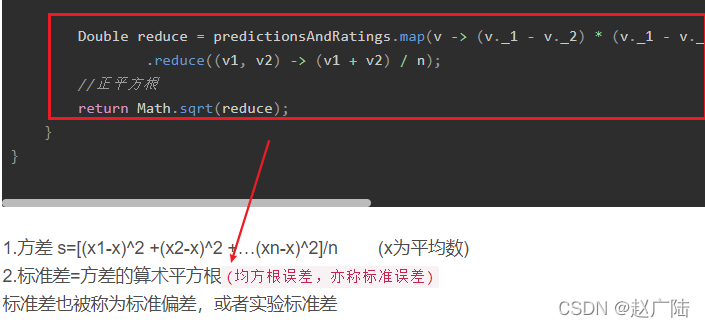1.方差 s=[(x1-x)^2 +(x2-x)^2 +…(xn-x)^2]/n 　　(x为平均数)

2.标准差=方差的算术平方根(均方根误差，亦称标准误差)

用户：943电影：1682评论：100000

RMSE(校验集) = 1.9042189155615398E-5, rank = 10, lambda = 0.01, numIter = 8
RMSE(校验集) = 4.241470253244084E-5, rank = 10, lambda = 0.01, numIter = 9
RMSE(校验集) = 1.4449138813397543E-5, rank = 10, lambda = 0.01, numIter = 10
RMSE(校验集) = 6.082115231352825E-5, rank = 11, lambda = 0.01, numIter = 8
RMSE(校验集) = 7.053700942035736E-5, rank = 11, lambda = 0.01, numIter = 9
RMSE(校验集) = 2.6551033361283012E-5, rank = 11, lambda = 0.01, numIter = 10
RMSE(校验集) = 3.31869548209156E-5, rank = 12, lambda = 0.01, numIter = 8
RMSE(校验集) = 7.16881674580905E-5, rank = 12, lambda = 0.01, numIter = 9
RMSE(校验集) = 4.8896811754448735E-5, rank = 12, lambda = 0.01, numIter = 10

789->1598: 9.396627380043618
789->1368: 8.14482648449507
789->1466: 7.38882286732828
789->1462: 6.81871292883074
789->1059: 6.795506701087147
789->337: 6.780524189917936
789->267: 6.772379061916583
789->1184: 6.688179558303117
789->906: 6.528331428606722
789->854: 6.466069010345999



• 总共有943个用户，1682个电影（已经去重），100000条评分数据；
• 如程序，我们把所有数据分为三部分：
• 60%用于训练、20%用于校验、20%用于测试模型；
• 模型在不同参数下的均方根误差（RMSE）值，以及对应的参数，最优的参数选择均方根误差（RMSE）最小的参数值—即最优参数模型建立；
• 使用20%的测试模型数据来测试模型的好坏，也就是均方根误差（RMSE），在最优参数模型基础上提升了81.34%的准确率。

SaaS 模式云数据仓库必修课|
2月前
|

119 2
|
2月前
|

25 0
|
3月前
|

82 1
|
3月前
|

118 0
|
3月前
|
JSON 分布式计算 大数据

97 0
|
3月前
|
canal 分布式计算 关系型数据库

83 0
|
3月前
|
SQL 分布式计算 大数据

105 0
|
3月前
|

22 0
|
3月前
|

35 0
|
3月前
|

28 0